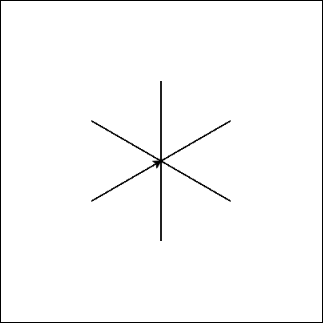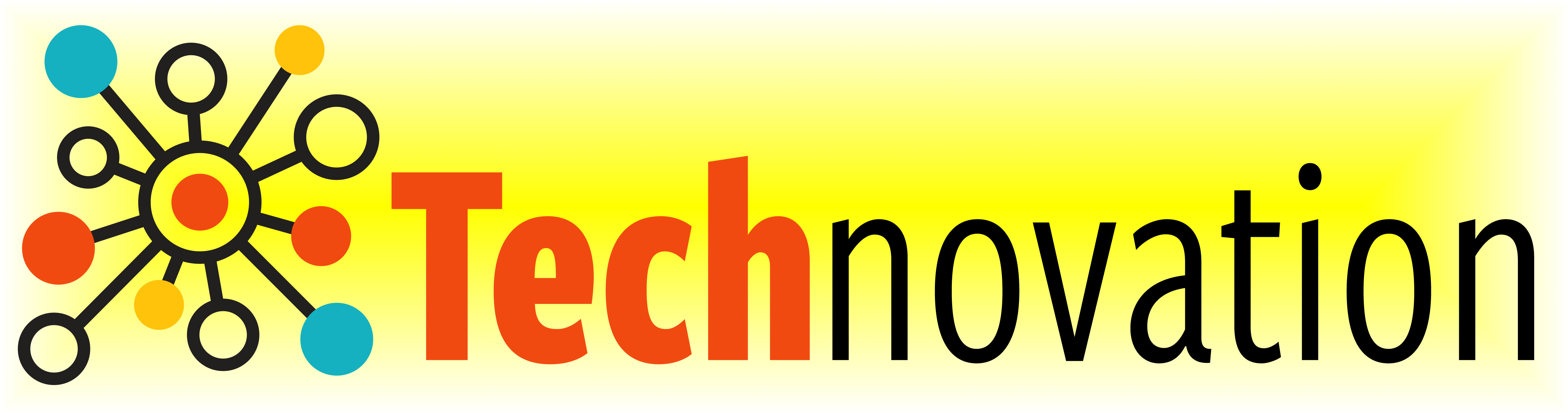# 3.5. Practice Makes Perfect¶

Turtle Commands

Command

What does it do?

forward( distance )

Move forward a specified distance

backward( distance )

Move backward a specified distance

left(90)

Turns 90 degrees to the left (you can use any angle, not just 90!)

right(90)

Turns 90 degrees to the right

circle( radius )

Draws a circle with the specified radius

goto( x, y )

Move straight to the position with coordinates (x, y). Note: the center is (0, 0)

up()

Stop leaving a trail

down()

Start drawing a trail

color( c )

Set the color to c (https://trinket.io/docs/colors)

for i in range( n ):

Repeat n times

If you don’t remember what these commands do, give them a try below!

Let’s take another look at drawing a square. How could we re-write it using a loop?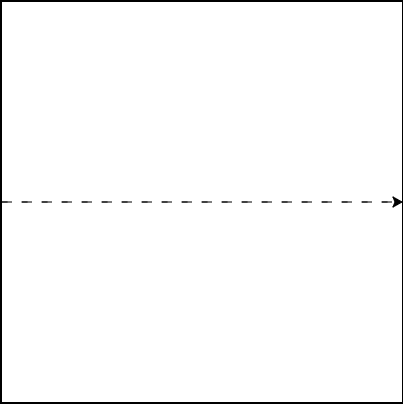Let’s use what we know about loops to draw a dashed line across the entire box!
The code below draws two dashes. See if you can modify it to use a loop!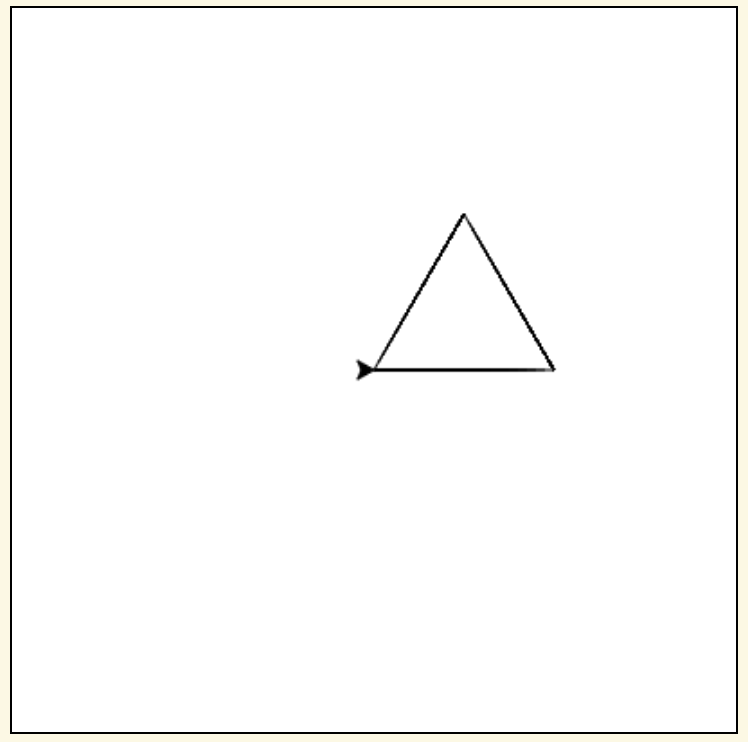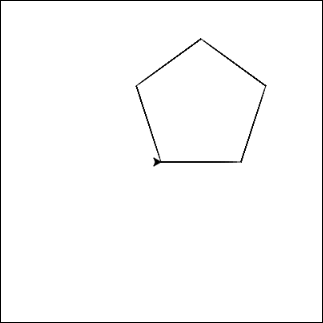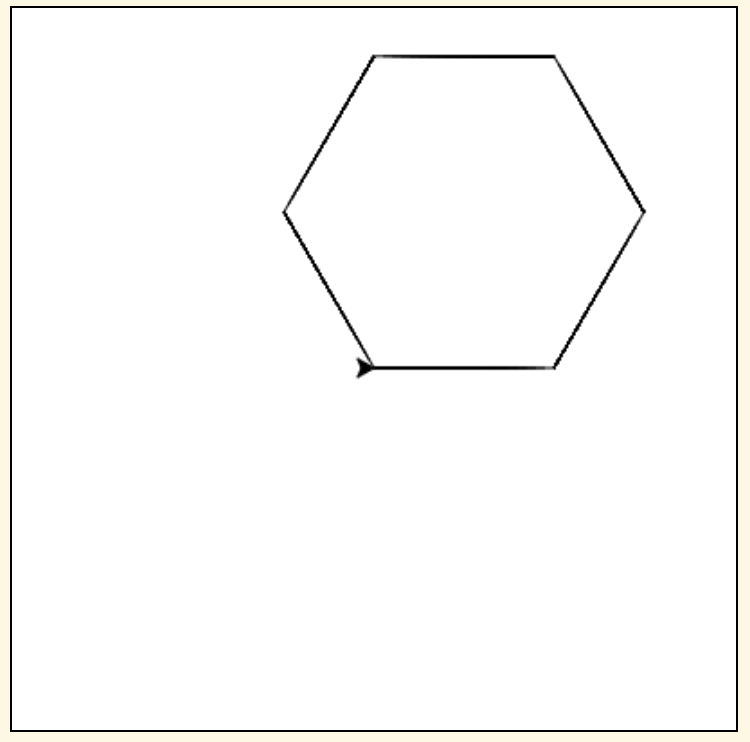Why stop with squares? Shapes with any number of sides can be drawn using a loop!
Start with your code that draws a square. Then, change the number of times the loop repeats and the angle that you turn each time to see if you can draw these shapes.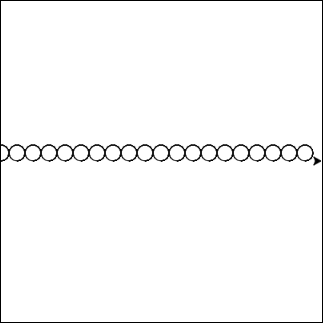Try converting you dashed line into a row of circles! Let’s draw 20 circles, each with a radius of 10.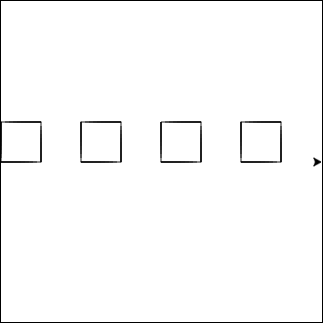Try drawing a row of squares!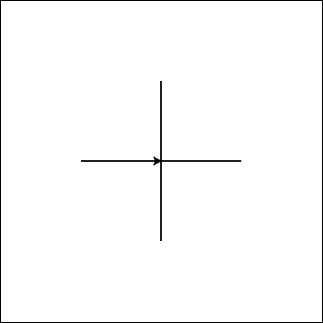Try drawing a plus sign using a loop!
If you’re not sure how to do it with a loop, try writing it out line by line first. Once you’ve got it drawing a plus sign, see if you can put any repeated code in a loop!